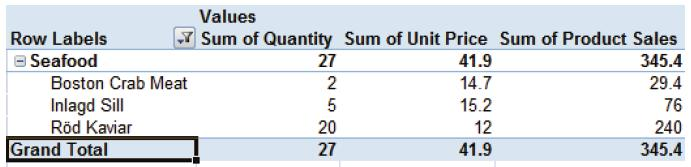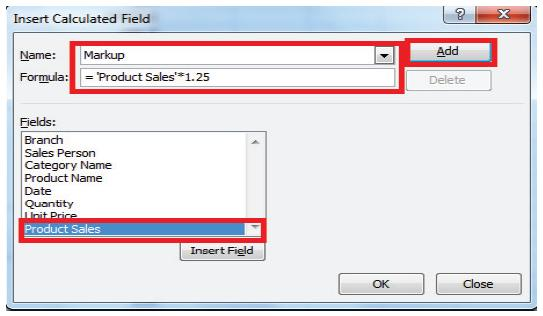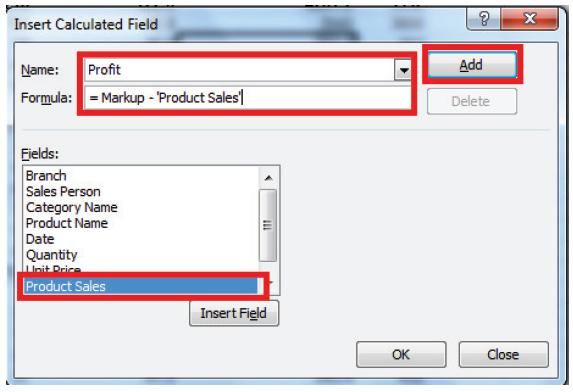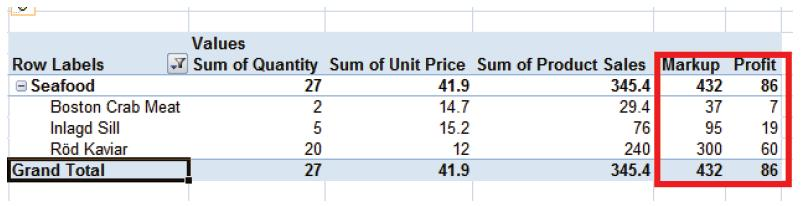# Business Automation and Software Blog

Excel Tips and Tricks / Business Reporting Question: How can I create my own calculated fields to include in the PivotTable? I intend to have a mark-up of 25% on the sales figure and then calculate the profit.

Answer: By using the PivotTable formulae option

Why: To create calculated fields

Applies To MS Excel 2003, 2007, 2010

1. This example is based on last week’s tip on how to create a pivotTable.

2. Select any cell in the PivotTable.3. Select as given below:

Excel 2007Excel 20104. The Insert a Calculated field window will open. Enter the following and select add.5. Rename the field on the PivotTable to Markup (double click on “sum of Markup” field, delete the words “sum of” and press enter).

6. Repeat the Process to calculate Profit.

7. Enter the following and select add.8. Select Ok.

9. Rename the field on the PivotTable to Profit,

10. The result will be as given below.The Markup and Profit figures have been computed by way of adding two calculated fields to the PivotTable.